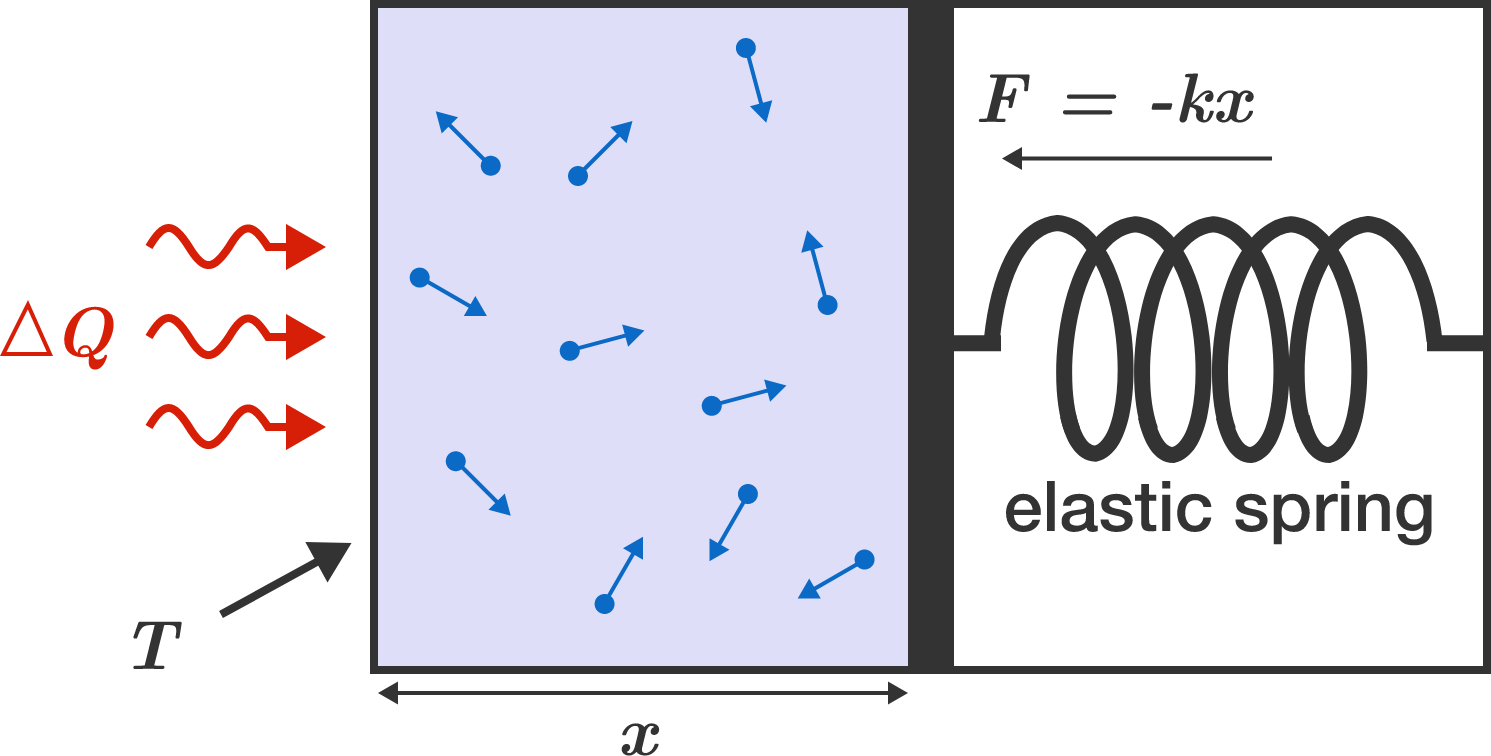# Gas in a piston

Helium gas is enclosed in a gas-tight piston which is held in position by the clamping force of an elastic spring. In mechanical equilibrium, the gas pressure $p = -\frac FA$ corresponds to the tensioning force $F$ of the spring divided by the area $A$. The gas is then brought to a temperature of $T = 600 \,\text{K}$ by rapid heating from room temperature $T = 300 \,\text{K}$.

What is the final temperature of the gas (in units of Kelvin) after setting a new mechanical balance with the spring?Details and Assumptions:

• The volume of the gas is $V = Ax,$ where $A$ is the cross-sectional area and $x$ is the piston position.
• The mechanical force of the spring equals $F = - k x,$ where $k$ is the spring constant.
• The piston does not move during the heating from $300 \,\text{K}$ to $600 \,\text{K}$.
• Helium can be treated as an ideal gas.
• Energy is transferred only by mechanical work. The piston is perfectly thermally insulated.
×

Problem Loading...

Note Loading...

Set Loading...